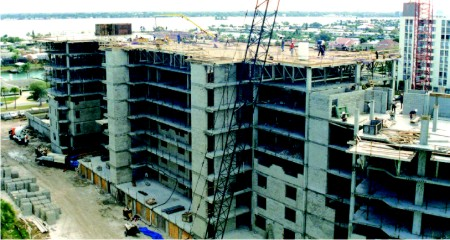Prabhat Kumar, Retd. Scientist, Central Building Research Institute and P.C. Sharma, Retd. Head Material Sciences, Structural Engineering Research Centre, Ghaziabad

Addition of fiber reinforcement in mortar and concrete provides a large number of benefits. The mathematical modeling of this new material is needed to exploit its benefits to fullest extent. This paper brings past and present trends to achieve this. A compact mathematical model is presented and the process of calibration of model is demonstrated. Results are encouraging.

## Introduction

Safety and economy are major concerns in building construction. The existing structures support safety aspects of the past and current design practices. However, economy and rationality aspects require continuous research in view of the advancements in material technology and more ambitious functional requirements of new structures. For example, high strength concrete (HSC) offers economic advantage in concrete filled tube columns, core walls, lower storey columns and pre–cast piles. Addition of silica fumes for strength enhancement makes HSC brittle. The economic advantage of HSC is partly off set on account of increased susceptibility to structural damage under abnormal operational conditions, which arise during natural disasters like earthquake and cyclone. Fibers may be added to HSC to compensate for the negative effect of silica fumes . This product is fiber reinforced concrete (FRC). The addition of fibers marginally affects pre–peak stress–strain response of concrete, however, the post–peak response changes substantially (See Figure 1).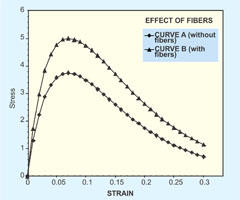Figure 1: Typical effects of fiber addition to concrete
• Compressive strength increases
• Peak strain increases
• The rate of drop of post–peak descending branch moderates
• Stress at large strain enhances substantially
Improved structural and strength properties of FRC, like impact and fatigue resistance, are fruitfully exploited in Fiber Reinforced Shotcrete , and polymer concrete, which is used in structural repair . Addition of fibers arrests formation and propagation of micro–cracks and improves tensile strength of concrete to delay its fracture.

Recent revolution in the digital computer hardware and software technology places tremendous computation power at the disposal of an analyst. It must be gainfully utilized in the design of structures such as pavement and runways in particular. At present, the material technology appears to be moving faster than the corresponding analytical developments [4–7]. This article presents recent developments in the analytical modeling of FRC. The possibilities which still remain are described and future extensions are proposed. Availability of a satisfactory mathematical model can promote greater utilization of benefits obtained by adding fiber to concrete and also enhance areas of application.

## Mechanics of Fiber Reinforcement of Concrete

There are a large number of variables in the problem of FRC.
1. Strength of the matrix to be reinforced,
2. Material of fibers–It may be steel, glass or polypropylene,
3. Aspect ratio of fibers l/f where l is length and f is diameter,
4. End profile of fibers–hooked, bent or straight,
5. Body profile of fibers–It may be twisted to improve bonding.
6. Amount of fiber. Volume fraction Vf and weight fraction wf define the amount of fibers. These are related as in Equation 1. The ratio of unit weights is approximately 3.25 for steel fiber.

wf = (Unit weight of fiber material/Unit weight of matrix) Vf (1)
Published experimental studies on FRC provide empirical expressions for prediction of stress–strain behavior. These expressions are derived by using boundary conditions of slope at the origin, peak point, inflexion point and at any other point. Some of the expressions consist of a single expression for entire curve whereas others may be in the form of two or more segments, applicable to pre– and post–peak, respectively.

The area under the stress–strain curve is taken as a measure of deformation energy absorbing capacity of a material. The toughness ratio (TR) is defined as the ratio of area under the actual stress–strain curve to the area under an ideal rigid plastic material of similar strength. A toughness index (TI) is also defined as the ratio of toughness of fiber reinforced matrix to that of the un–reinforced matrix. It may be seen that TR is always less than unity while TI is always greater than unity. An integration of Equation of stress–strain curve is required to calculate toughness index. Ductility, on the other hand, is the ability of the material to deform without failure. The shape and rate of decay of descending branch of stress–strain curve characterize ductility property of a material. Toughness and ductility properties are important when loading involves impact, repetition and reversal.

It has been found  that everything being equal, the improvement due to fiber addition was relatively more pronounced in lower matrix compressive strengths. To facilitate further mathematical development, reinforcing index (RI) has been introduced. Experimental observations revealed  that higher RI resulted in smaller the slope of the post–peak branch of stress–strain curve. Therefore, the area under the stress–strain curve increased. RI can be defined with respect to volume fraction Vf as (VRI = Vf l/Φ) as well as with respect to weight fraction as (WRI = wf l/Φ). These two shall be related as in Equation 1. It was found  that VRI in excess of 2.0 resulted in deterioration rather than enhancement of properties. It indicates that there is an optimum benefit that can be derived from fiber addition. This may occur due to difficulty in ensuring uniform distribution of fibers in the body of concrete element.

## Mathematical Modeling of FRC

In the derivation of analytical expression for stress–strain curve of FRC, following expressions are most commonly used.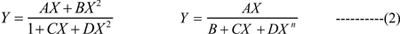A, B, C and D in Equation 2 are parameters which are determined from the boundary conditions at origin, peak point, inflexion point and at any other suitable location. The number of boundary conditions must be equal to the number of parameters. This mathematical fact limits the number of parameters which can occur in a general expression. A mathematical model is meaningless unless all its parameters can be characterized from the measurable quantities. In this presentation stress and strain are denoted by f and ε, respectively. Further, Y = fc/fP, X = εcP. Subscript P applies at peak point while c is a general point. Following studies highlight the difficulties in arriving at a suitable expression for stress–strain curve of FRC.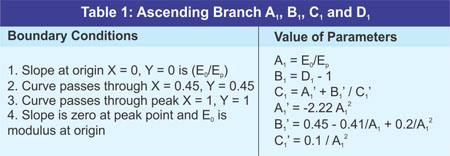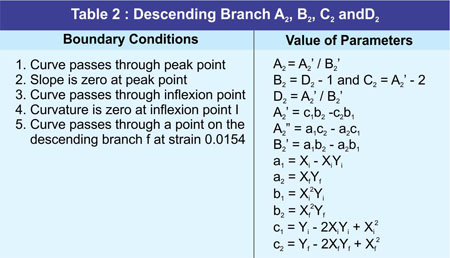Fanella and Naaman  described properties of FRC through Equations [3–5]. Starting with the first expression of Equation 2 values for parameters A to D are as given in Tables 1 and 2.

fcf'= 1.9 RI + fc' --------------------------------(3)

Ecf= 162 fcf'+ 8270 ----------------------------(4)

εP= 0.00079RI + 0.0041 (fcf'/ fc') --------------(5)

Mebarkia and Vipulanandan  used second expression of Equation 2 as Equation 3.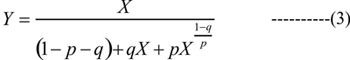This expression satisfies the boundary conditions that it passes through origin and peak point. The following additional boundary conditions were used to determine parameters p and q (1) At origin initial tangent modulus is Eo/Ep(2) Slope is zero at peak point. Parameters p and q must meet the followings additional conditions p + q > 0; p + q 0. Through regression analysis with the experimental observations, the following model was produced. Xf and XP are fiber and polymer contents by weight, respectively. An integration of Equation 3 is required to calculate toughness index.

q = 1 – p – Ep/ Eo and

p = A + BXf+ CXf2 -------------(4)

Where,

A = – 0.36 + 0.076XP– 0.0029XP2

B = 0.46 – 0.068XP+ 0.0023XP2

C= 0.0055 + 0.0013XP– 0.000070XP2

Ezeldine and Balaguru  also used second expression of Equation 2 as Equation 5. One should note that Equations 3 and 5 are identical [â = (1–q)/p]. This model was calibrated for hooked as well as straight fibers with reinforcing index by weigh as RI and ri, respectively.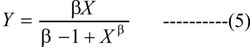Hooked fibers:

β = 1.093 + 0.074*10-4Eo and

Eo= 9.610*104[RI]-0.926

Straight fibers:

β = 1.093 + 7.4818 [ri]-1.387

Mansur et al  used second expression of Equation 2 in his study. Equation 6 was used for the descending branch of stress–strain curve. The ascending branch used k1= 1 and k2= 1. This experimental program used hooked steel fibers.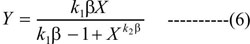For confined non–fiber concrete, k1= 2.77(ρsfy/fo) and k2= 2.19 (ρsfy/fo ) + 0.17

For confined fiber concrete, k1= 3.33 (ρsfy/fo ) + 0.12 and k2= 1.62 (ρsfy/fo ) + 0.35

Here ρs is the volumetric ratio of lateral ties, fy is proof stress of lateral ties and fo is unconfined strength of concrete.

Complete details of above mathematical models of FRC can be found in the original papers. The presentation of this section shows that analytical modeling of FRC is important and that use of empirical techniques is rampant in this endeavor. Also, the available analytical models are complicated and suffer on account of limited validity. More research effort is obviously required.

## Recent Developments

Barr and Lee  deviated from the prevailing trend of using either of the expressions given in Equation 2 to propose use of a double exponential model. In this approach, two exponential functions are subtracted to obtain proper shape of the stress–strain curve. Smith and Young  first used exponential function for this purpose (Equation 7). The double–exponential model uses Equation 8. This double–exponential model can be further developed by assigning values to parameters C1, C2 and C3 from the boundary conditions of FRC problem.

Y = X EXP(1 – X) ----------------------------(7)

Y = C1[EXP(–C2X) – EXP(–C3X)] -----------(8)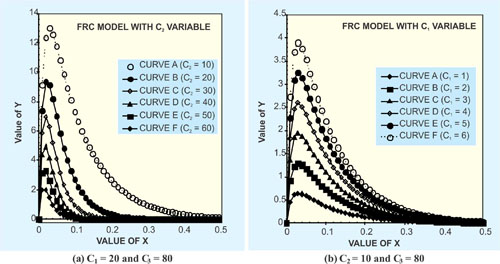Figure 2: Results of parametric study (continued)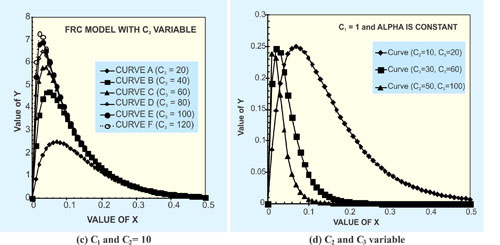Figure 2: Results of parametric study (concluded)

The first exponential function dominates the tail end where as the second expression influences the shape of stress–strain curve in the initial part. A parametric study of Equation 8 (Figure 2) shows that different combination of parameters yields a family of stress–strain curves. It should be possible to use this formulation for mathematical modeling of stress–strain behavior of FRC. This model is applied to the published experimental data on steel fibers . The experimental and analytical results are compared in Figures 3 and 4.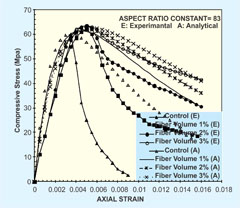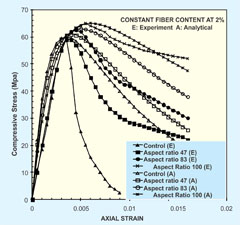Figure 3: Modeling of FRC with constant aspect ratio Figure 4: Modeling of FRC with constant volume fraction

Compact model  is also available in which the analytical line is made to pass through a point located on the tail of stress–strain curve. Equations 9 to 12 describe this model.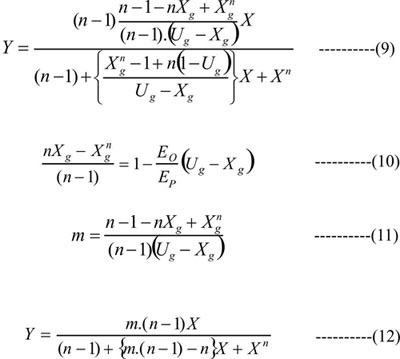Equation 9 is the stress–strain relation in which solution of Equation 10 provides the value of exponent n. Equation 9 is obtained from Equation 12 in which value of m is as given in Equation 11. This model is also tried and the tail end point is taken at strain of 0.015. This model is applied to the published experimental data on steel fibers . The experimental and analytical results are compared in Figures 5 and 6.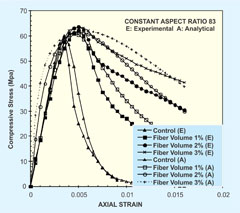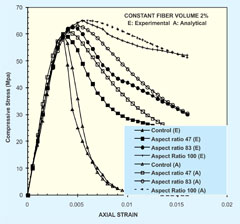Figure 5: Modeling of FRC with constant aspect ratio Figure 6: Modeling of FRC with constant volume fraction

X and Y are non-dimensional strain and stress, respectively; n = Model Parameter; m is a derived quantity (equation 11); U is a derived quantity (Xg/Yg). Subscript g denotes general point; O defines quantity at origin; and P applies to quantity at peak point. The application of compact model is more satisfactory than the double exponential model.

## Conclusion

Stress–strain behavior of Fiber Reinforced Concrete is analytically modeled in this paper. Two published analytical models, which were never applied in modeling of FRC, have been used in the present study. Some difficulties were faced in the process. First of all, it was difficult to read the values of experimental observations from the published documents. Secondly, the exact conditions of experimental investigations were not known.Practically all experimental investigators propose an analytical model which applies very well to their data. It is not surprising because, the analytical models are based on the regression analysis of their own data.

This paper considers data of other investigators, so this effort is unique. The effort of analytical modeling reported in this paper is very encouraging. This procedure shall be refined further and shall be reported in subsequent publications on this topic. More experimental data must be generated. For example, all varieties of fibers must be considered. The analytical procedure should then be applied to this more extensive experimental data to develop general expressions for toughness index etc. The proposed models are more suitable for analytical treatment like integration etc.

## References

• Ezeldine, A. S. and Balaguru, P. N. (1992) "Normal and high strength fiber reinforced concrete under compression," Journal of Materials in Civil Engineering (ASCE), V. 4(4), pp. 415–429.
• Barrett, S. and McGreath, D. R. (1995) "Shotcrete support design in blocky ground," Tunneling and Underground Space Technology, V. 10(1), pp. 79–89.
• Mabarkia, S. and Vipulanandan, C. (1992) "Compressive behavior of glass fiber reinforced mortar," J of Materials in Civil Engineering (ASCE), V. 4(1), pp. 91–105.
• Shah, S. P. and Naaman, A. E. (1976) "Mechanical properties of glass and steel fiber reinforced mortar," ACI Journal, V. 73(1), pp. 50 – 53.
• Hughes, B. P. and Fattuhi, N. I. (1977) "Stress–strain curves for fiber reinforced concrete in compression," Cement and Concrete research, V. 7(2), pp. 173–183.
• Fanella, D. A. and Naaman, A. E. (1985) "Stress–strain properties of fiber reinforced mortar in compression," ACI Journal, V. 82(4), pp. 475–483.
• Mansur, M. A., Chin, M. S. and Wee, T. H. (1997) "Stress–strain relationship of confined high strength plain and fiber concrete," Journal of Materials in Civil Engineering (ASCE), 9(4), pp. 171–178.
• Barr, B. and Lee, M. K., (2003) "Modeling the strain softening behavior of plain concrete using a double–exponential model," Magazine of Concrete research, 55 (4), pp. 343–353.
• Smith, G. M. and Young, L. E. (1956) "Ultimate Flexural Analysis Based on Stress–Strain Curve of Cylinder." ACI Journal, Proc. V. 53 (6), pp. 597–609.
• Kumar, P. (2004) A compact analytical model for unconfined concrete under uni axial compression. Materials and Structures (RILEM), V. 37(9), pp. 585–590.
Concrete is a heterogeneous composite complex material, and its hardened property is influenced by its fresh property. Concrete today has transformed into an advanced type with new and innovative ingredients added - either singly or in
ICRETE offers many benefits apart from reducing cement content and giving high grades saving to ready-mix concrete companies; it helps reduce shrinkage and permeability in concrete slabs, increases the durability of concrete, and also works
UltraTech Cement Limited, India’s largest cement and ready-mix concrete (RMC) company, and Coolbrook, a transformational technology and engineering company, will jointly develop a project to implement Coolbrook’s RotoDynamic HeaterTM (RDH)
Plastic shrinkage cracking occurs when fresh concrete is subjected to a very rapid loss of moisture. It is caused by a combination of factors such as air and concrete temperature, relative humidity, and wind velocity at the surface of concrete. These can cause
This paper covers the research work carried out on cement plastering process for internal and external building wall by using spray plastering machine. Objective of study is to experiment and compare the plastering activity by conventional way and
In recent years, the speed of construction has increased very fast; buildings which used to take 3-5 years are now getting completed in 1-2 years. There is a race to complete projects faster, but due to this speedy construction, the quality of construction is often
With its zero-emission characteristics, hydrogen has become a promising decarbonization path for the cement industry. While there are several issues that need to be resolved in the use of hydrogen, there are also many advantages, so much so that the growth
Reinforced concrete is a composite material which is made using concrete and steel bars. Concrete takes the compressive forces and steel bar takes tensile forces. Concrete around the steel bar protects it from corrosion by providing an alkaline environment
Amol Patil, Sr. Specialist - General Manager (Admixture and Specialty Products), Master Builders Solutions (India), and Nilotpol KAR, Managing Director, Master Builders Solutions (South Asia), present a paper on the concept of low viscosity concrete in
Cement companies are constantly innovating to meet global sustainability standards and improve logistics, shelf life, and utility of cement, while reducing wastage. Thei aim is to reduce their environmental impact without compromising their product
Researchers at the Indian Institute of Technology Madras have developed a treatment process using solar thermal energy to recycle construction and demolition debris. Waste concrete from demolition was heated using solar radiation to produce recycled concrete
As a new-age innovative building material, TRC is especially suited for maintenance of existing structures, for manufacturing new lightweight precast members, or as a secondary building material to aid the main building material. Textile Reinforced Concrete
The day is not far for the adoption of this innovative, eco-friendly, and cost-effective bottom ash – concrete process technology by construction agencies undertaking road/infrastructure project works, real estate developers, ready mix concrete (RMC) operators
Using headed bars instead of hooked bars offer several advantages like requirement of reduced development length, less congestion, ease of transport and fixing at site, better concrete consolidation, and better performance under seismic loads.
It can be said that ever since the publication of the document of World Commission on Environment and Development , the focus of the world has diverted towards sustainability. Gro Harlem Bruntland  defined sustainable development as “development
The effects of shrinkage, creep, crack-width, and deflection in concrete are often ignored by designers while designing structural members. These effects, if not considered in some special cases such as long span slabs or long cantilevers, may become very
Relief shelve walls are a unique concept that use only conventional construction materials like PCC / RCC / steel reinforcements, and work on a completely different fundamental to resist the lateral load caused due to soil. Information on the various dimensions
Increasing energy costs, overcapacity, and environmental pollution are the top concerns of the cement industry, which is one of the major contributors to CO2 emissions. Dr S B Hegde, Professor, Department of Civil Engineering, Jain College of Engineering
Betolar, a Finnish start-up, and innovator of geopolymer concrete solution Geoprime®, has expanded its operations to Europe and Asian markets including India, Vietnam and Indonesia. Betolar’s innovation Geoprime® is the next-generation, low carbon
It is estimated that in India each million clay bricks consume about 200 tons of coal and emit around 270 tons of CO2; on the other hand, with fly ash bricks production in an energy-free route, there are no emissions. Dr. N. Subramanian, Consulting

### II&TW

To get latest updates on whatsapp, Save +91 93545 87773 and send us a 'Saved' message